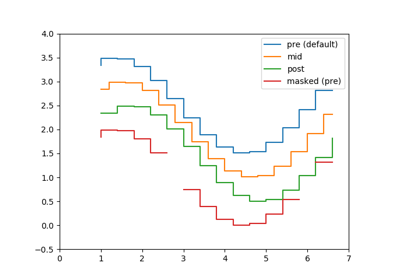# matplotlib.pyplot.step¶

matplotlib.pyplot.step(x, y, *args, **kwargs)[source]

Make a step plot.

Call signatures:

step(x, y, [fmt], *, data=None, where='pre', **kwargs)
step(x, y, [fmt], x2, y2, [fmt2], ..., *, where='pre', **kwargs)


This is just a thin wrapper around plot which changes some formatting options. Most of the concepts and parameters of plot can be used here as well.

Parameters: x : array_like 1-D sequence of x positions. It is assumed, but not checked, that it is uniformly increasing. y : array_like 1-D sequence of y levels. fmt : str, optional A format string, e.g. 'g' for a green line. See plot for a more detailed description. Note: While full format strings are accepted, it is recommended to only specify the color. Line styles are currently ignored (use the keyword argument linestyle instead). Markers are accepted and plotted on the given positions, however, this is a rarely needed feature for step plots. data : indexable object, optional An object with labelled data. If given, provide the label names to plot in x and y. where : {'pre', 'post', 'mid'}, optional, default 'pre' Define where the steps should be placed: 'pre': The y value is continued constantly to the left from every x position, i.e. the interval (x[i-1], x[i]] has the value y[i]. 'post': The y value is continued constantly to the right from every x position, i.e. the interval [x[i], x[i+1]) has the value y[i]. 'mid': Steps occur half-way between the x positions. lines A list of Line2D objects representing the plotted data. **kwargs Additional parameters are the same as those for plot.

Notes

Note

In addition to the above described arguments, this function can take a data keyword argument. If such a data argument is given, the following arguments are replaced by data[<arg>]:

• All arguments with the following names: 'x', 'y'.

## Examples using matplotlib.pyplot.step¶Step Demo